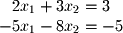Given a system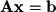, a unique solution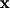exists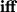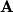is invertible. This solution can be found using the following rule: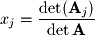where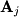is the matrixwith the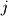th column replaced by the constant vector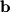.
Excercise 5-2.
Solve the following system using Cramer's rule: